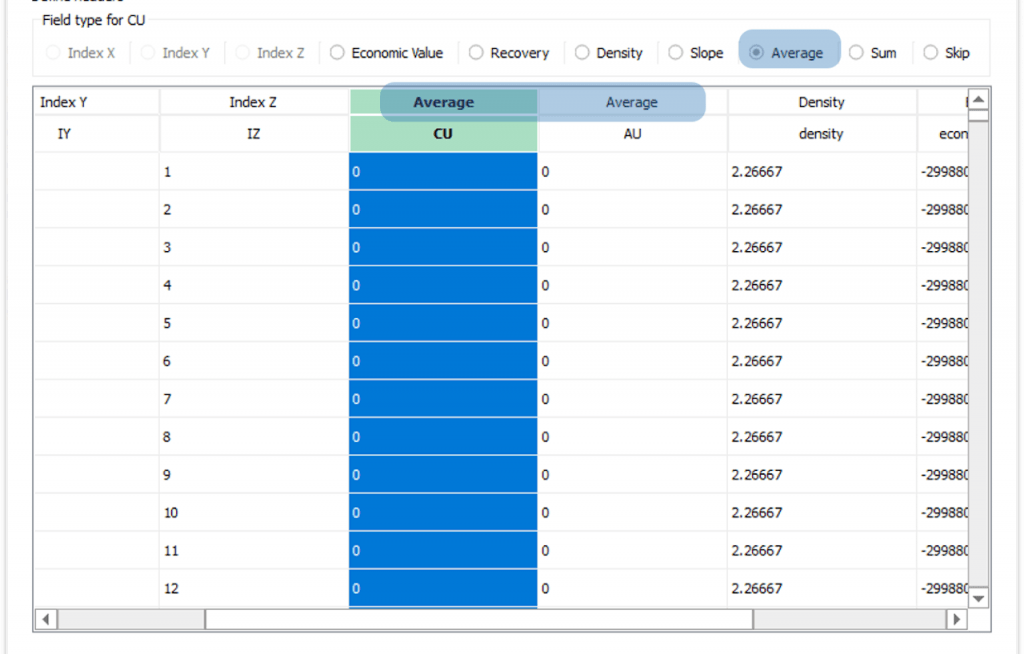# Refining the NPV upper bound

After having identified the NPV upper bound of your project, it is recommended that you start adding average constraints, sum constraints, and stockpiling. If there are any average and sum variables in your project, they will be already imported at this stage. Nonetheless, you can always define new variables in the calculator if necessary. Next, you can see some examples of average constraints, sum constraints, and stockpiling being employed in the Marvin dataset.

## Average constraints

Average constraints are based on quantifiable parameters modeled block by block. To use this feature, the dataset must include an auxiliary field/column representing the desired limit for each block.

#### When to use?

Average constraints are usually employed for blending low-grade and high-grade blocks to enhance profitability. Nonetheless, they have a diverse range of applications, being applicable to any variable that can be modeled based on these assumptions.

#### Set up

The figures below depict two parameters imported as average variables.The limits of average variables can be defined in the Scenario tab. As depicted below, it is possible to input limits (A), for each period range (B), weights (C) and each destination (D).

You in Overview tab you can see these limits as depicted below.

#### Example

The scenario below exemplifies the impact of an Average constraint on the Super Best Case of the Marvin dataset. In this case, the NPV goes from 942 M$NPV in the Super Best Case to 933 M$ in the new scenario with Average constraints. Hence, the new constraint has only a small impact on the NPV.

On the left, CU grade with average constraint (0.42 min and 0.46 max) , and on the right CU grade with no average constraint.

On the left, Cumulative NPV (933 M$) with CU grade constrained between 0.42 and 0.46. On the right, CU grade unconstrained (942 M$).

## Sum constraints

Sum constraints are based on the sum of any quantifiable parameter modeled block by block. To use this feature, the dataset must include an auxiliary field/column representing the desired limit for each block.

#### When to use?

Sum constraints controls the total amount mined in a single period of the respective sum variable for each block. Basically, any variable which needs to have a total amount controlled could be employed. Some examples of variables that could be controlled with a Sum constraint are listed below:

• Total amount of processing hours
• Tonnages and proportions of rock type and metal production.
• Consumption of inputs such as energy spent during comminution, and fleet hours spent to mobilize material.
• Contaminants control on the processing plant during each period.

The user can define:

• Different sum limits for each material.
• Different sum limits for each interval.
• Different sum limits for each destination.
• Combine all the options above in order to achieve globally optimized results.

#### Set up

Sum variables can be constrained in a similar manner to average ones. The figures below depict one parameter imported as a sum variable and its respective limits in the Sum tab.

#### Example

Consider the scenario above with a maximum limit of 13.000 hours of processing equipment. This constraint can be inserted at the Sum tab previously depicted. The impact of this restriction can be evaluated when results are compared to a unrestricted scenario.

On the left, processing hours with sum constraint (13000 max). On the right, unconstrained processing hours.

On the left, cumulative NPV (931 M$) with the sum of Proc hours constrained at 13,000 max. On the right, Proc hours unconstrained (942 M$).

The resulting reports show the 13000 hours limit was respected for each period. However, the NPV decreased from 942 M$NPV in the Super Best Case to 931 M$ in this new defined scenario. Such a fall demonstrates that in order to achieve the full value of the project, identified in the Super Best Case, the addition of more processing equipment would be necessary. This addition might not be feasible or reasonable, showing that, in this case, the new results are closer to a more realistic scenario.

## Stockpilling

Stockpiles are optimized blocks that were initially designated for disposal. They are processed after the main optimization phase, where the algorithm analyzes their value (Revenue – Fixed Mining Cost – Rehandling Cost) compared to the cost of discarding them. If worthy, blocks will be reclaimed, complementing production shortfalls over time.

#### Set up

To enable the stockpiles on the interface the first step is on the General tab where two inputs are required:

1. Fixed Mining Cost: value used to decompose the economic value while considering stockpiles;

2. Rehandling Cost: represents the cost to reclaim blocks from the stockpile to the process

After that, on the Destinations tab, you can define stockpile limits for each processing plant added, remembering that this limit is based on the life of mine, not in a period time frame.

NoteSince stockpiles are a post-processing unit of the algorithm, total tonnage restrictions might be violated when the stockpiles are processed. Total tonnage restrictions are only considered during the processing unit of the algorithm.